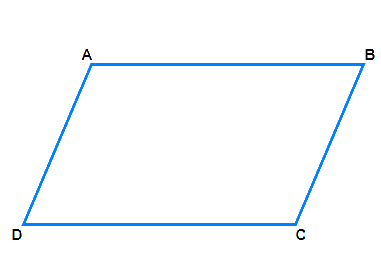# Ex.3.3 Q3 Understanding Quadrilaterals Solution-Ncert Maths Class 8

## Question

Can a quadrilateral $$ABCD$$ be a parallelogram if

(i) $$∠D +∠B = 180^\circ?$$

(ii) $$AB = DC = 8 \rm \,cm, AD = 4 \rm \,cm$$ and $$BC = 4.4 \rm \,cm?$$

(iii) $$∠A = 70^\circ$$ and  $$∠C = 65^\circ?$$Video Solution
Understanding Quadrilaterals
Ex 3.3 | Question 3

## Text Solution

i) What is Known?

Given figure is a quadrilateral

What is Unknown?

If $$ABCD$$ is a parallelogram when $$\angle {\rm{D }} + \angle {\rm{B }} = {\rm{18}}0^\circ ?$$

Reasoning:

A parallelogram is a quadrilateral whose opposite sides are parallel.

In parallelogram opposite angles are equal and adjacent angles are supplementary.

Using this property, we can calculate the unknown angles.

Steps:

Using the angle sum property of a quadrilateral,

\begin{align}\angle A+\angle B+\angle D+\angle C&={{360}^{\circ}} \\\angle A+\angle C+{{180}^{\circ}}&={{360}^{\circ}} \\\angle A+\angle C&={{360}^{\circ}}-{{180}^{\circ}} \\\ \angle A+\angle C&={{180}^{\circ}}\end{align}

(Opposite angles should also be of same measures.)

For $$\angle {\rm{D}} + \angle {\rm{B}}$$ $$= {\rm{18}}0^\circ$$, is a parallelogram.

If the following conditions is fulfilled, then $$ABCD$$ is a parallelogram.

The sum of the measures of the adjacent angles should be $$180^\circ$$.

Opposite angles should also be of same measure.

ii) What is Known?

Given figure is a quadrilateral.

What is Unknown?

$$ABCD$$ be a parallelogram if $$AB = DC = 8 \rm \,cm, AD = 4 \rm \,cm$$ and $$BC = 4.4 \rm \,cm$$

Reasoning:

A parallelogram is a quadrilateral whose opposite sides are parallel.

Steps:

Property of parallelogram: The opposite sides of a parallelogram are of equal length. Opposite sides $$AD$$ and $$BC$$ are of different lengths. So, it’s not parallelogram.

iii) What is Known?

Given figure is a quadrilateral.

What is Unknown?

$$ABCD$$ be a parallelogram if $$\angle {\rm{A }} = {\rm{ 7}}0^\circ {\rm{ }}$$ and $$\angle {\rm{C }} = {\rm{ 65}}^\circ ?$$

Reasoning:

A parallelogram is a quadrilateral whose opposite sides and angles are equal.

Steps:

Property: In a parallelogram opposite angles are equal.

So, $$\angle {\rm{A }} = {\rm{ 7}}0^\circ {\rm{ }}$$and $$\angle {\rm{C }} = {\rm{ 65}}^\circ {\rm{ }}$$are not equal.

So $$ABCD$$ is not parallelogram.

Learn from the best math teachers and top your exams

• Live one on one classroom and doubt clearing
• Practice worksheets in and after class for conceptual clarity
• Personalized curriculum to keep up with school